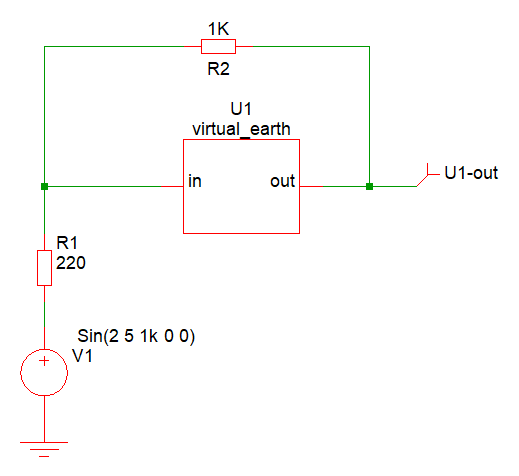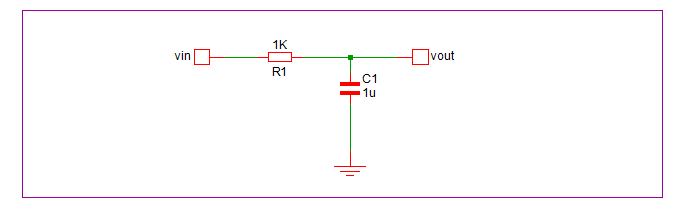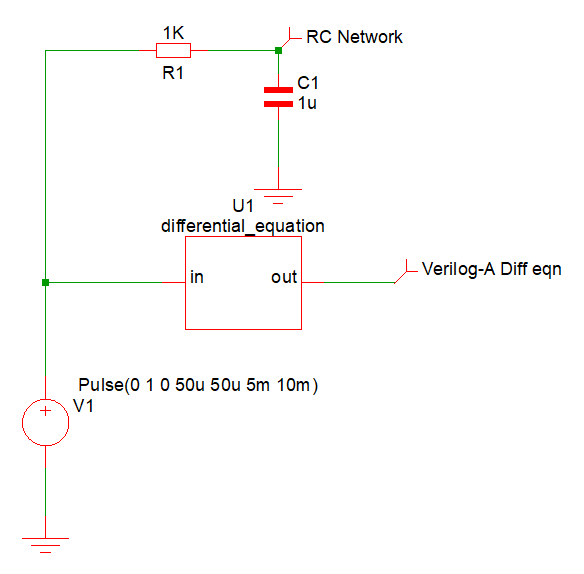# Indirect Assignments

Indirect assignments are a way of defining an equation to be solved. Here we present two examples of indirect assignments, a perfect virtual earth and the solution of a first order differential equation.

In this topic:

## Virtual Earth

Consider this example of an indirect branch assignment:

V(out) : V(in) == 0.0 ;

This can be expressed in words as "drive V(out) so that V(in) equals zero". This creates a virtual earth at vin.

Here is the complete Verilog-A module:

'include "disciplines.vams"

module virtual_earth(in, out) ;

electrical in, out;

analog
begin

V(out) : V(in) == 0.0 ;

end
endmodule
The above can be connected in the following circuit:Differential Equation
the in node of U1 will be maintained at exactly zero volts therefore fabricating an ideal inverting amplifier with a gain of 1k/220.

## Differential Equation

Consider this Verilog-A module:
'include "disciplines.vams"

module differential_equation(in, out) ;

electrical in, out;

analog
begin

V(out) : ddt(V(out)) == (V(in) - V(out))/1m ;

end
endmodule

This solves the differential equation:

$\displaystyle \frac{d.vout}{dt} = \frac{(vin - vout)}{0.001}$

This is the equation that governs the following circuit:You can try this with this schematic: